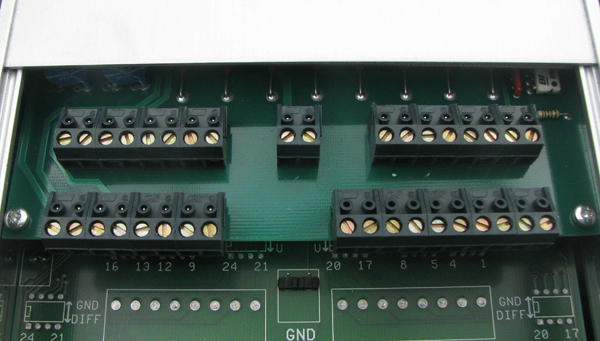Support / FAQ / PC-logger / Divide the digital inputs

### Divide the digital inputsA PC-logger with a digital IN/OUT has eight digital inputs and four digital outputs,  except for the PC-logger 2100 that has three digital/pulse counter inputs where one input can be used as a digital output. The digital inputs and outputs are presented in EasyView in the form of an integer. This integer represents a binary value and can be divided, using the formulas below, so that each digital input and output is shown as a single curve in the graph.

Here is the formula for dividing the digital inputs:

SET DigIn = ID31
GRAPH "DigIn 1"(Din) = DigIn BIT 0
GRAPH "DigIn 2"(Din) = DigIn BIT 1
GRAPH "DigIn 3"(Din) = DigIn BIT 2
GRAPH "DigIn 4"(Din) = DigIn BIT 3
GRAPH "DigIn 5"(Din) = DigIn BIT 4
GRAPH "DigIn 6"(Din) = DigIn BIT 5
GRAPH "DigIn 7"(Din) = DigIn BIT 6
GRAPH "DigIn 8"(Din) = DigIn BIT 7

Here is the formula for dividing the digital outputs:

SET DigOut = ID32
GRAPH "DigOut 1"(Dout) = DigOut BIT 0
GRAPH "DigOut 2"(Dout) = DigOut BIT 1
GRAPH "DigOut 3"(Dout) = DigOut BIT 2
GRAPH "DigOut 4"(Dout) = DigOut BIT 3